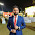# Arithmetic Progression C Program

In mathematics, an arithmetic progression (AP) or arithmetic sequence is a sequence of numbers such that the difference between the consecutive terms is constant. For instance, the sequence 5, 7, 9, 11, 13, 15 … is an arithmetic progression with common difference of 2.
If the initial term of an arithmetic progression is$a_1$ and the common difference of successive members is d, then the nth term of the sequence ($a_n$) is given by:$\ a_n = a_1 + (n - 1)d,$
Source: wikipedia

#### C Program to print Arithmetic Progression:

```#include <stdio.h>

int main()
{
int i, term, difference, ap;
printf("Enter number of terms: ");
scanf("%d",&term);
printf("Enter the common difference of Arithmatic Progression: ");
scanf("%d",&difference);
printf("The arithmatic progression from 1 to %d with term difference %d is: \n",term,difference);
for(i=1;i<=term;i++){
ap = 1+(i-1)*difference;
printf("%d ",ap);
}
return 0;
}
```
Share:

#### 4 comments :

1.All the best. It was helpful to me. Thanks. carry on, Sakib.

2.Thanks,helped a lot.

3.b-( :-# =p~ \$-) (b) (f) x-) (k) (h) (c) cheer

4.This blog awesome and i learn a lot about programming from here.The best thing about this blog is that you doing from beginning to experts level.

Love from

Spam comments will be deleted. :)